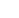# Tópico: Criando Sistema de Reborn (2Moons/Dekaron)

1. ##Criando Sistema de Reborn (2Moons/Dekaron)

Bom aqui vai um tuto básico de como adicionar sistema de reborn (reset)
em servidores de 2Moons/Dekaron. Então vamos ao que interessa.

*****************************************
************ DK Reborn System ************
*****************************************

@ Escrito por: lolpol
@ Modificado por: Janvier123
@ Tradução/Revisão por: daddycool.

****************************************
************ Server Side Setup ************
****************************************

1) Abra/Inicie: "SQL Query Analyzer"
2) Logue-se com seu Login e Senha do SQL
3) Copie e cole o código abaixo:

Código:
```USE [character];
GO

ALTER TABLE [user_character] ADD [Reborn] [smallint] DEFAULT(0) NOT NULL;```

4) Clique em "Parse Query" (Ctrl + F5) e depois clique em "Execute Query" (F5)
5) Feche o SQL Query Analyzer:
6) Abra/Inicie: "Enterprise Manager"
7) Vá para: Console Root/Microsoft SQL Servers/SQL Server Group/(local) (Windows NT)/Managemnt/SQL Server Agent/Jobs
8) Clique com o botão direito na janela a direita e escolha "New Job"
9) Na guia "General" em "Name:" coloque "Reborn" ou o que você quiser
Na guia "Steps" clique em "New"
"Step name:" coloque "AutoReborn"
"Type:" selecione "Transact-SQL Script (TSQL)"
"Database:" selecione "Character"
"Command:" copie e cole o código abaixo:

Código:
```USE [character];
GO

UPDATE dbo.user_character
SET wLevel = 1, dwExp = 0, wStatPoint = 250 * (Reborn + 1), nHP=106, nMP=16, dwMoney = dwMoney - (50000000), wStr = 6, wDex = 3, wCon = 4, wSpr = 2, wPosX = 336, wPosY = 366, Reborn = Reborn + 1, wMapIndex = 7, wSkillPoint = 0
WHERE (byPCClass = 0) AND (wlevel >= 170) AND (dwMoney >= (50000000)) AND (Reborn < 50);

UPDATE dbo.user_character
SET wLevel = 1, dwExp = 0, wStatPoint = 250 * (Reborn + 1), nHP=106, nMP=16, dwMoney = dwMoney - (50000000), wStr = 6, wDex = 3, wCon = 4, wSpr = 2, wPosX = 336, wPosY = 366, Reborn = Reborn + 1, wMapIndex = 7, wSkillPoint = 0
WHERE (byPCClass = 1) AND (wlevel >= 170) AND (dwMoney >= (50000000)) AND (Reborn < 50);

UPDATE dbo.user_character
SET wLevel = 1, dwExp = 0, wStatPoint = 250 * (Reborn + 1), nHP=106, nMP=16, dwMoney = dwMoney - (50000000), wStr = 6, wDex = 3, wCon = 4, wSpr = 2, wPosX = 336, wPosY = 366, Reborn = Reborn + 1, wMapIndex = 7, wSkillPoint = 0
WHERE (byPCClass = 2) AND (wlevel >= 170) AND (dwMoney >= (50000000)) AND (Reborn < 50);

UPDATE dbo.user_character
SET wLevel = 1, dwExp = 0, wStatPoint = 250 * (Reborn + 1), nHP=106, nMP=16, dwMoney = dwMoney - (50000000), wStr = 6, wDex = 3, wCon = 4, wSpr = 2, wPosX = 336, wPosY = 366, Reborn = Reborn + 1, wMapIndex = 7, wSkillPoint = 0
WHERE (byPCClass = 3) AND (wlevel >= 170) AND (dwMoney >= (50000000)) AND (Reborn < 50);

UPDATE dbo.user_character
SET wLevel = 1, dwExp = 0, wStatPoint = 250 * (Reborn + 1), nHP=106, nMP=16, dwMoney = dwMoney - (50000000), wStr = 6, wDex = 3, wCon = 4, wSpr = 2, wPosX = 336, wPosY = 366, Reborn = Reborn + 1, wMapIndex = 7, wSkillPoint = 0
WHERE (byPCClass = 4) AND (wlevel >= 170) AND (dwMoney >= (50000000)) AND (Reborn < 50);

UPDATE dbo.user_character
SET wLevel = 1, dwExp = 0, wStatPoint = 250 * (Reborn + 1), nHP=106, nMP=16, dwMoney = dwMoney - (50000000), wStr = 6, wDex = 3, wCon = 4, wSpr = 2, wPosX = 336, wPosY = 366, Reborn = Reborn + 1, wMapIndex = 7, wSkillPoint = 0
WHERE (byPCClass = 5) AND (wlevel >= 170) AND (dwMoney >= (50000000)) AND (Reborn < 50);

UPDATE dbo.user_character
SET wLevel = 1, dwExp = 0, wStatPoint = 250 * (Reborn + 1), nHP=106, nMP=16, dwMoney = dwMoney - (50000000), wStr = 6, wDex = 3, wCon = 4, wSpr = 2, wPosX = 336, wPosY = 366, Reborn = Reborn + 1, wMapIndex = 7, wSkillPoint = 0
WHERE (byPCClass = 6) AND (wlevel >= 170) AND (dwMoney >= (50000000)) AND (Reborn < 50);```
Clique em "Parse", depois "Apply" e "Ok"

10) Na guia "Schedules" clique em "New Schedule"
11) "Name:" colque "Schedule1" ou o que quiser
"Schedule Type" selecione "Recurring"
Clique em "Change" e coloque o seguinte:

Occurs: Daily
Daily: Every 1 Day(s)
Daily Frequency: Occurs Every: 1 Minute(s)
Starting at: 0:00:00
Ending at: 23:59:59
Duration: (today's date)
No end Date

12) Clique em "Ok"
13) Crie outra Job
14) Na guia "General" em "Name:" coloque "Reset_Login_Flag" ou o que você quiser
Na guia "Steps" clique em "New"
"Step name:" coloque "Login_Flag"
"Type:" selecione "Transact-SQL Script (TSQL)"
"Database:" selecione "Account"
"Command:" copie e cole o código abaixo:

Código:
```USE [account];
GO

UPDATE dbo.user_profile
SET login_flag = 0
WHERE (login_flag = 1100);```
Clique em "Parse", depois "Apply" e "Ok"

15) Na guia "Schedules" clique em "New Schedule"
16) "Name:" colque "Schedule2" ou o que quiser
"Schedule Type" selecione "Recurring"
Clique em "Change" e coloque o seguinte:

Occurs: Daily
Daily: Every 1 Day(s)
Daily Frequency: Occurs Every: 1 Minute(s)
Starting at: 0:00:00
Ending at: 23:59:59
Duration: (today's date)
No end Date

17) Clique em "Ok"
18) Inicie todas as Jobs.

Pronto, seu sistema de Reborn está pronto.Responder com Citação

#### Informações de Tópico

##### Usuários Navegando neste Tópico

Há 1 usuários navegando neste tópico. (0 registrados e 1 visitantes)

####Permissões de Postagem

• Você não pode iniciar novos tópicos
• Você não pode enviar respostas
• Você não pode enviar anexos
• Você não pode editar suas mensagens
•